# 使用深度学习增加图像分辨率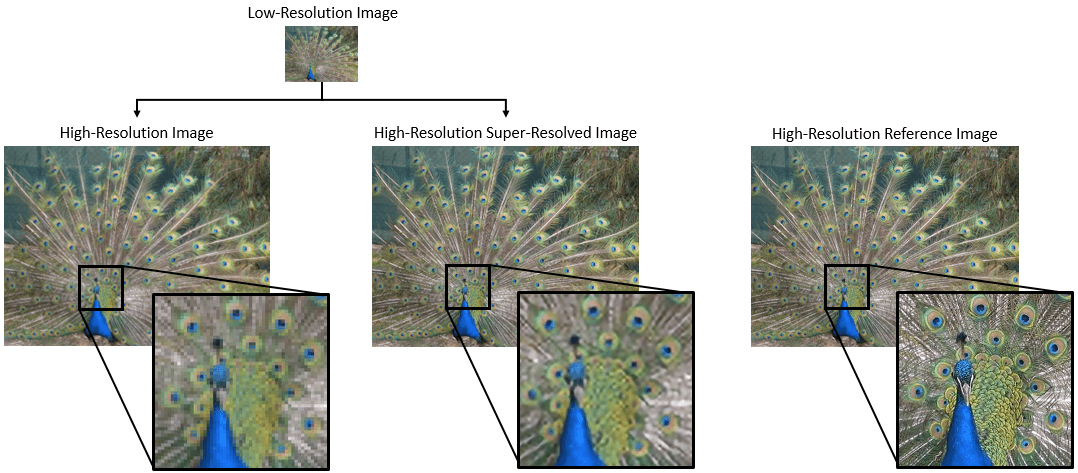### VDSR 网络

VDSR 是一种用于执行单图像超分辨率  的卷积神经网络架构。VDSR 网络学习低分辨率图像和高分辨率图像之间的映射。这种映射是有可能获得的，因为低分辨率和高分辨率图像具有相似的图像成分，主要区别在于高频细节。

VDSR 采用一种残差学习策略，这意味着网络会学习估计残差图像。在超分辨率中，残差图像是高分辨率参考图像和低分辨率图像之间的差异，低分辨率图像已使用双三次插值扩增以匹配参考图像的大小。残差图像包含关于图像高频细节的信息。

VDSR 网络基于彩色图像的亮度检测残差图像。图像的亮度通道 `Y` 使用红色、绿色和蓝色像素值的线性组合来表示每个像素的亮度。另一方面，图像的两个色度通道 CbCr 使用红色、绿色和蓝色像素值的其他线性组合来表示色差信息。VDSR 仅使用亮度通道进行训练，因为人类对亮度变化的感知比对颜色变化更敏感。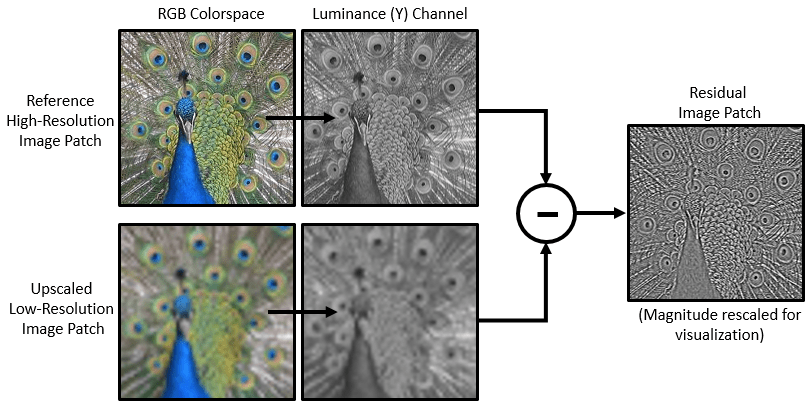### 下载训练和测试数据

```dataDir =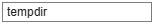tempdir; downloadIAPRTC12Data(dataDir);```

```trainImagesDir = fullfile(dataDir,"iaprtc12","images","02"); exts = [".jpg",".bmp",".png"]; pristineImages = imageDatastore(trainImagesDir,FileExtensions=exts);```

`numel(pristineImages.Files)`
```ans = 616 ```

### 准备训练数据

```upsampledDirName = trainImagesDir+filesep+"upsampledImages"; residualDirName = trainImagesDir+filesep+"residualImages";```

• 将图像转换为 YCbCr 颜色空间

• 以不同缩放因子缩小亮度 (Y) 通道，以创建低分辨率样本图像，然后使用双三次插值将图像大小调整为原始大小

• 计算原始图像和调整大小后的图像之间的差异。

• 将调整大小后的图像和残差图像保存到磁盘。

```scaleFactors = [2 3 4]; createVDSRTrainingSet(pristineImages,scaleFactors,upsampledDirName,residualDirName);```

### 定义训练集的预处理流程

```upsampledImages = imageDatastore(upsampledDirName,FileExtensions=".mat",ReadFcn=@matRead); residualImages = imageDatastore(residualDirName,FileExtensions=".mat",ReadFcn=@matRead);```

```augmenter = imageDataAugmenter( ... RandRotatio=@()randi([0,1],1)*90, ... RandXReflection=true);```

```patchSize = [41 41]; patchesPerImage = 64; dsTrain = randomPatchExtractionDatastore(upsampledImages,residualImages,patchSize, ... DataAugmentation=augmenter,PatchesPerImage=patchesPerImage);```

```inputBatch = preview(dsTrain); disp(inputBatch)```
``` InputImage ResponseImage ______________ ______________ {41×41 double} {41×41 double} {41×41 double} {41×41 double} {41×41 double} {41×41 double} {41×41 double} {41×41 double} {41×41 double} {41×41 double} {41×41 double} {41×41 double} {41×41 double} {41×41 double} {41×41 double} {41×41 double} ```

### 设置 VDSR 层

VDSR 有 20 个卷积层，因此感受野和图像补片大小为 41×41。图像输入层接受具有一个通道的图像，因为仅使用亮度通道训练 VDSR。

```networkDepth = 20; firstLayer = imageInputLayer([41 41 1],Name="InputLayer",Normalization="none");```

```convLayer = convolution2dLayer(3,64,Padding=1, ... WeightsInitializer="he",BiasInitializer="zeros",Name="Conv1");```

`relLayer = reluLayer(Name="ReLU1");`

```middleLayers = [convLayer relLayer]; for layerNumber = 2:networkDepth-1 convLayer = convolution2dLayer(3,64,Padding=[1 1], ... WeightsInitializer="he",BiasInitializer="zeros", ... Name="Conv"+num2str(layerNumber)); relLayer = reluLayer(Name="ReLU"+num2str(layerNumber)); middleLayers = [middleLayers convLayer relLayer]; end```

```convLayer = convolution2dLayer(3,1,Padding=[1 1], ... WeightsInitializer="he",BiasInitializer="zeros", ... NumChannels=64,Name="Conv"+num2str(networkDepth));```

`finalLayers = [convLayer regressionLayer(Name="FinalRegressionLayer")];`

`layers = [firstLayer middleLayers finalLayers];`

### 指定训练选项

```maxEpochs = 100; epochIntervals = 1; initLearningRate = 0.1; learningRateFactor = 0.1; l2reg = 0.0001; miniBatchSize = 64; options = trainingOptions("sgdm", ... Momentum=0.9, ... InitialLearnRate=initLearningRate, ... LearnRateSchedule="piecewise", ... LearnRateDropPeriod=10, ... LearnRateDropFactor=learningRateFactor, ... L2Regularization=l2reg, ... MaxEpochs=maxEpochs, ... MiniBatchSize=miniBatchSize, ... GradientThresholdMethod="l2norm", ... GradientThreshold=0.01, ... Plots="training-progress", ... Verbose=false);```

### 训练网络

```doTraining =false; if doTraining net = trainNetwork(dsTrain,layers,options); modelDateTime = string(datetime("now",Format="yyyy-MM-dd-HH-mm-ss")); save("trainedVDSR-"+modelDateTime+".mat","net"); else load("trainedVDSRNet.mat"); end```

### 使用 VDSR 网络执行单图像超分辨率

• 基于高分辨率参考图像创建一个示例低分辨率图像。

• 使用双三次插值对该低分辨率图像执行 SISR，双三次插值是一种不依赖于深度学习的传统图像处理解决方案。

• 使用 VDSR 神经网络对该低分辨率图像执行 SISR。

• 以可视化方式比较使用双三次插值和 VDSR 重构的高分辨率图像。

• 量化超分辨率图像与高分辨率参考图像的相似性，以此评估前者的质量。

### 创建示例低分辨率图像

```fileNames = ["sherlock.jpg","peacock.jpg","fabric.png","greens.jpg", ... "hands1.jpg","kobi.png","lighthouse.png","office_4.jpg", ... "onion.png","pears.png","yellowlily.jpg","indiancorn.jpg", ... "flamingos.jpg","sevilla.jpg","llama.jpg","parkavenue.jpg", ... "strawberries.jpg","trailer.jpg","wagon.jpg","football.jpg"]; filePath = fullfile(matlabroot,"toolbox","images","imdata")+filesep; filePathNames = strcat(filePath,fileNames); testImages = imageDatastore(filePathNames);```

`montage(testImages)`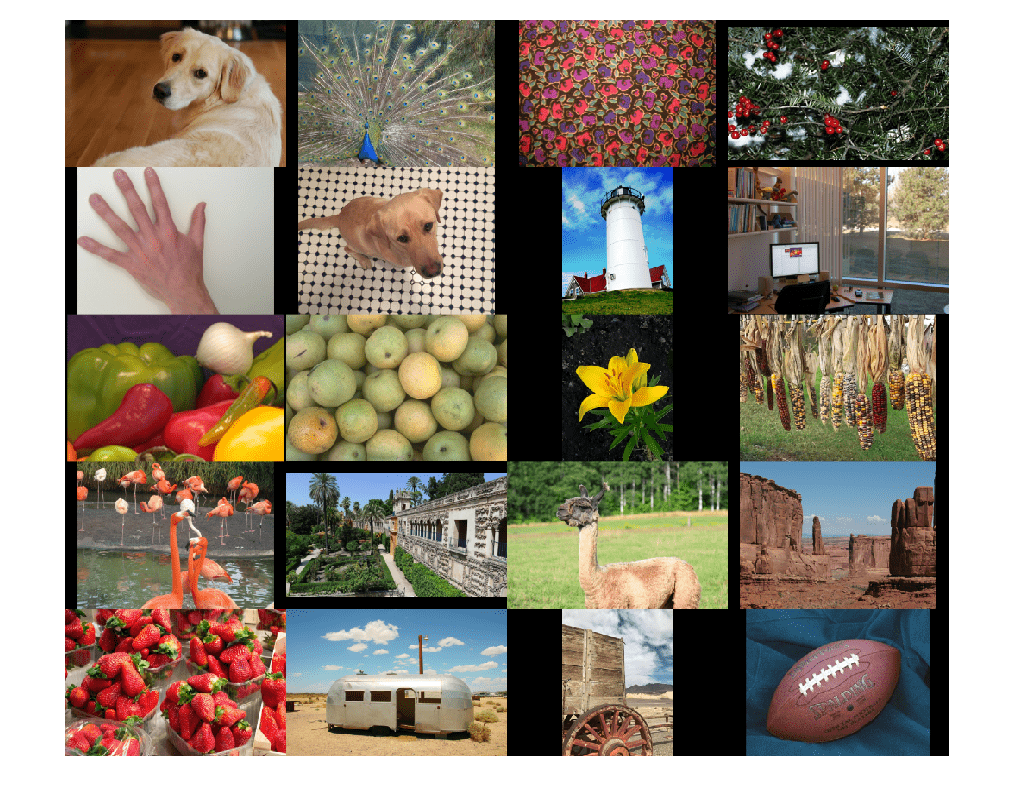```testImage =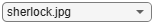"sherlock.jpg"; Ireference = imread(testImage); Ireference = im2double(Ireference); imshow(Ireference) title("High-Resolution Reference Image")```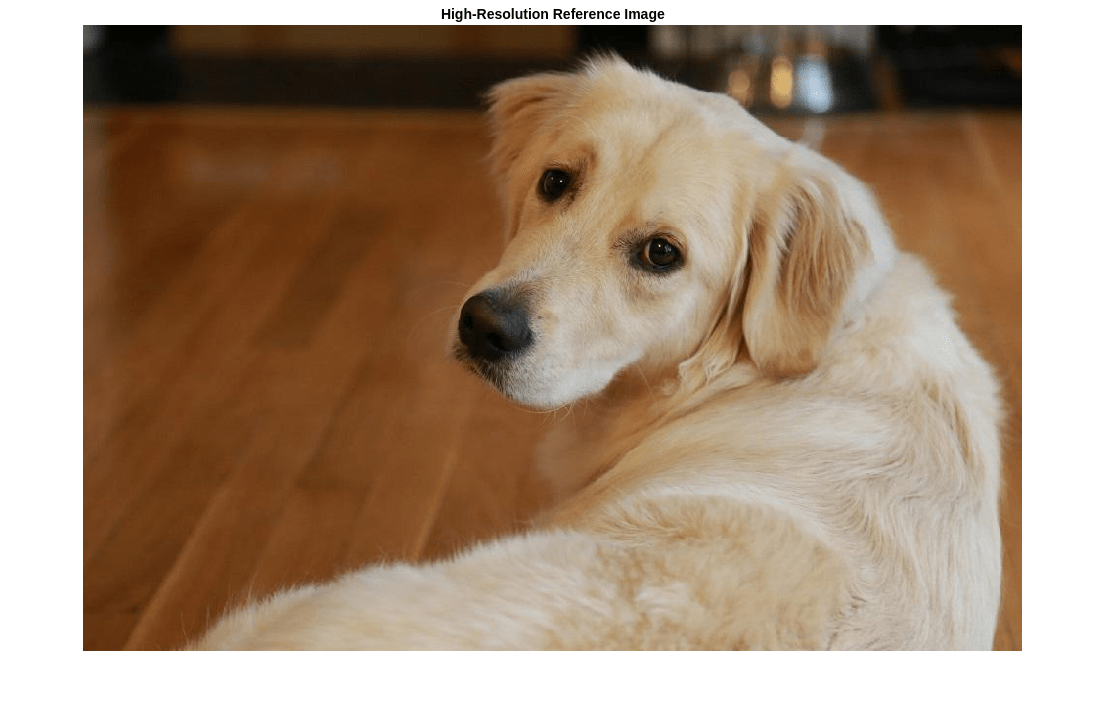```scaleFactor = 0.25; Ilowres = imresize(Ireference,scaleFactor,"bicubic"); imshow(Ilowres) title("Low-Resolution Image")```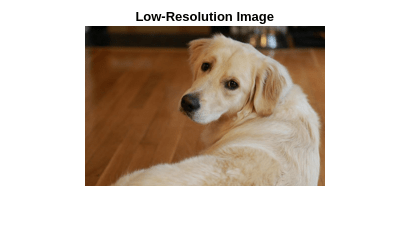### 使用双三次插值提高图像分辨率

```[nrows,ncols,np] = size(Ireference); Ibicubic = imresize(Ilowres,[nrows ncols],"bicubic"); imshow(Ibicubic) title("High-Resolution Image Obtained Using Bicubic Interpolation")```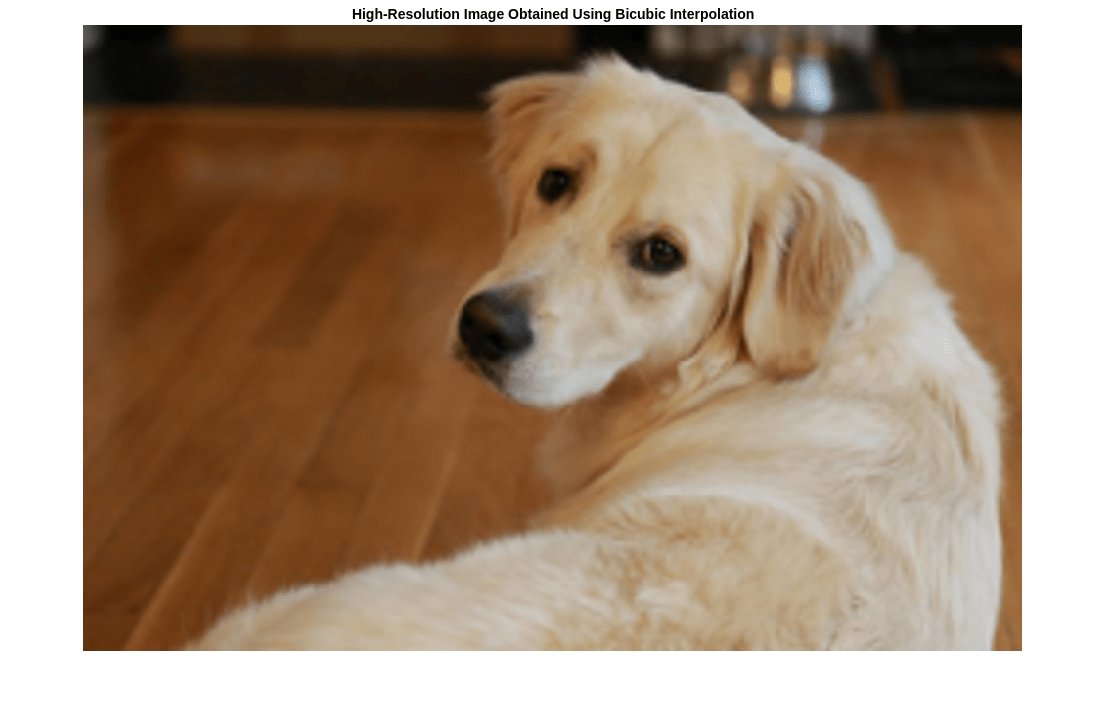### 使用预训练的 VDSR 网络提高图像分辨率

```Iycbcr = rgb2ycbcr(Ilowres); Iy = Iycbcr(:,:,1); Icb = Iycbcr(:,:,2); Icr = Iycbcr(:,:,3);```

```Iy_bicubic = imresize(Iy,[nrows ncols],"bicubic"); Icb_bicubic = imresize(Icb,[nrows ncols],"bicubic"); Icr_bicubic = imresize(Icr,[nrows ncols],"bicubic");```

```Iresidual = activations(net,Iy_bicubic,41); Iresidual = double(Iresidual); imshow(Iresidual,[]) title("Residual Image from VDSR")```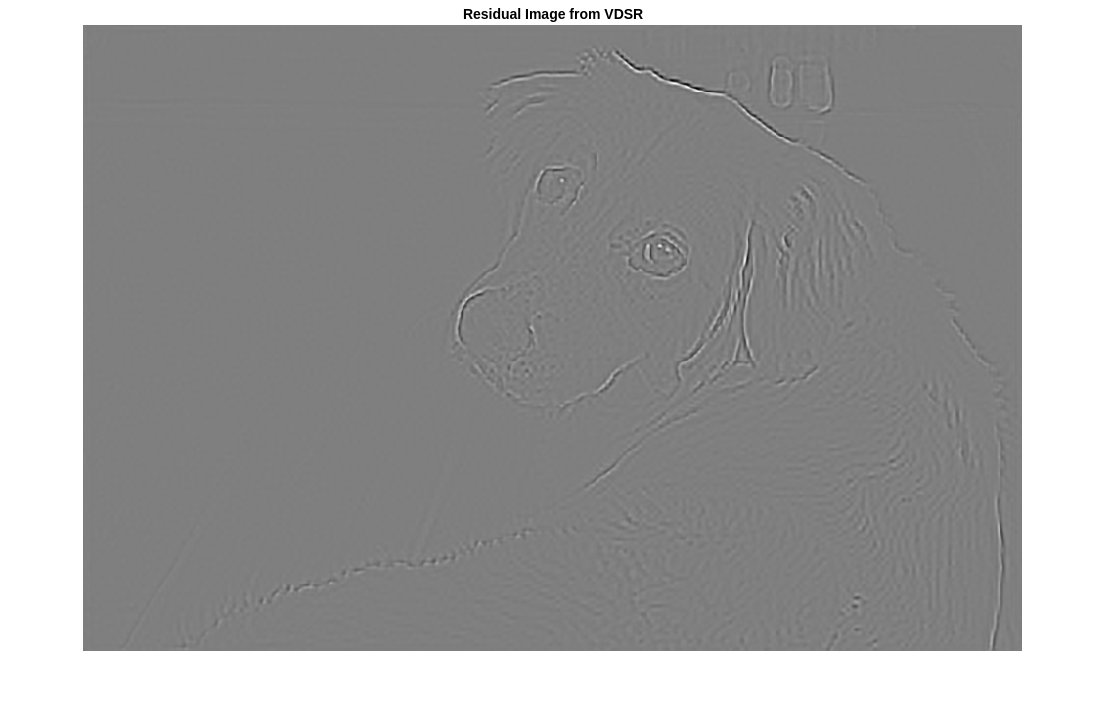`Isr = Iy_bicubic + Iresidual;`

```Ivdsr = ycbcr2rgb(cat(3,Isr,Icb_bicubic,Icr_bicubic)); imshow(Ivdsr) title("High-Resolution Image Obtained Using VDSR")```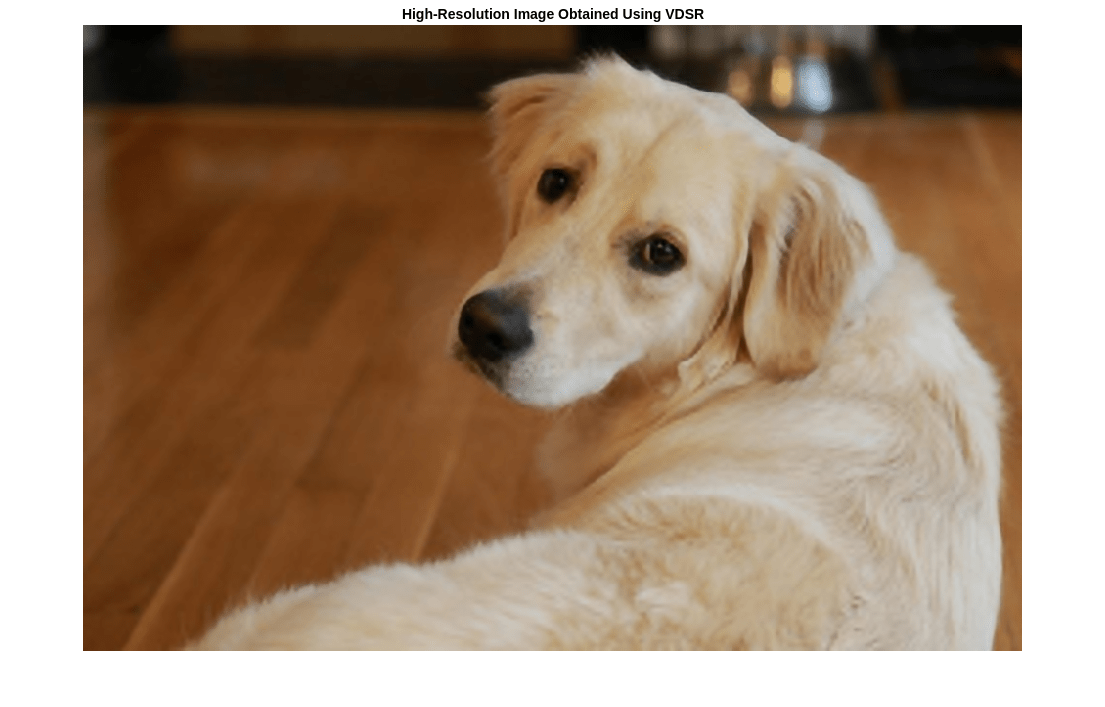### 可视化和定量比较

`roi = [360 50 400 350];`

```montage({imcrop(Ibicubic,roi),imcrop(Ivdsr,roi)}) title("High-Resolution Results Using Bicubic Interpolation (Left) vs. VDSR (Right)");````bicubicPSNR = psnr(Ibicubic,Ireference)`
```bicubicPSNR = 38.4747 ```
`vdsrPSNR = psnr(Ivdsr,Ireference)`
```vdsrPSNR = 39.2346 ```

`bicubicSSIM = ssim(Ibicubic,Ireference)`
```bicubicSSIM = 0.9861 ```
`vdsrSSIM = ssim(Ivdsr,Ireference)`
```vdsrSSIM = 0.9874 ```

`bicubicNIQE = niqe(Ibicubic)`
```bicubicNIQE = 5.1721 ```
`vdsrNIQE = niqe(Ivdsr)`
```vdsrNIQE = 4.7612 ```

```scaleFactors = [2 3 4]; vdsrMetrics(net,testImages,scaleFactors);```
```Results for Scale factor 2 Average PSNR for Bicubic = 31.467070 Average PSNR for VDSR = 31.481973 Average SSIM for Bicubic = 0.935820 Average SSIM for VDSR = 0.947057 Results for Scale factor 3 Average PSNR for Bicubic = 28.107057 Average PSNR for VDSR = 28.430546 Average SSIM for Bicubic = 0.883927 Average SSIM for VDSR = 0.894634 Results for Scale factor 4 Average PSNR for Bicubic = 27.066129 Average PSNR for VDSR = 27.846590 Average SSIM for Bicubic = 0.863270 Average SSIM for VDSR = 0.878101 ```

### 参考资料

 Kim, J., J. K. Lee, and K. M. Lee."Accurate Image Super-Resolution Using Very Deep Convolutional Networks."Proceedings of the IEEE® Conference on Computer Vision and Pattern Recognition.2016, pp. 1646-1654.

 Grubinger, M., P. Clough, H. Müller, and T. Deselaers."The IAPR TC-12 Benchmark:A New Evaluation Resource for Visual Information Systems."Proceedings of the OntoImage 2006 Language Resources For Content-Based Image Retrieval.Genoa, Italy.Vol. 5, May 2006, p. 10.

 He, K., X. Zhang, S. Ren, and J. Sun."Delving Deep into Rectifiers:Surpassing Human-Level Performance on ImageNet Classification."Proceedings of the IEEE International Conference on Computer Vision, 2015, pp. 1026-1034.